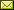## Observational Equivalence Using Schedulers for Quantum Processes

 Kazuya Yasuda (The University of Tokyo) Takahiro Kubota (The University of Tokyo) Yoshihiko Kakutani (The University of Tokyo)

 In the study of quantum process algebras, researchers have introduced different notions of equivalence between quantum processes like bisimulation or barbed congruence. However, there are intuitively equivalent quantum processes that these notions do not regard as equivalent. In this paper, we introduce a notion of equivalence named observational equivalence into qCCS. Since quantum processes have both probabilistic and nondeterministic transitions, we introduce schedulers that solve nondeterministic choices and obtain probability distribution of quantum processes. By definition, the restrictions of schedulers change observational equivalence. We propose some definitions of schedulers, and investigate the relation between the restrictions of schedulers and observational equivalence.

In Bob Coecke, Ichiro Hasuo and Prakash Panangaden: Proceedings of the 11th workshop on Quantum Physics and Logic (QPL 2014), Kyoto, Japan, 4-6th June 2014, Electronic Proceedings in Theoretical Computer Science 172, pp. 191–203.
Published: 28th December 2014.

 ArXived at: http://dx.doi.org/10.4204/EPTCS.172.13 bibtex PDF
References in reconstructed bibtex, XML and HTML format (approximated).
 Comments and questions to:eptcs@eptcs.org For website issues:webmaster@eptcs.org